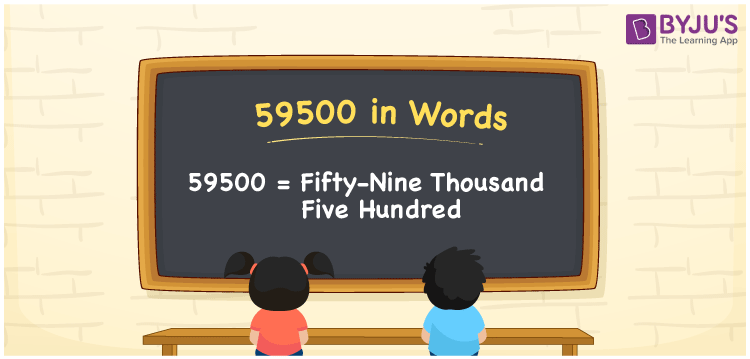# 59500 in Words

59500 in words is written as Fifty-Nine Thousand Five Hundred. Hence, the name of the number 59500 in English is Fifty-Nine Thousand Five Hundred. 59500 is a cardinal number. To indicate the currency equal to 59500, we can write it in words as Rupees Fifty-Nine Thousand Five Hundred. It is necessary to learn numbers in words using a place value chart for ease of understanding.

 59500 in Words Fifty-Nine Thousand Five Hundred Fifty-Nine Thousand Five Hundred in numerical form 59500

## 59500 in English Words

Generally, the English alphabet is used to express numbers in words. Thus, we can read and write the number 59500 in English as Fifty-Nine Thousand Five Hundred.## How to Write 59500 in Words?

The below table represents the place value chart with five columns as 59500 is a five-digit number

 Ten Thousand Thousands Hundreds Tens Ones 5 9 5 0 0

Hence, we can write the expanded form as:

5 x Ten Thousand + 9 x Thousand + 5 x Hundred + 0 x Ten + 0 x One

= 5 x 10000 + 9 x 1000 + 5 x 100 + 0 x 10 + 0 x 1

= 50000 + 9000 + 500 + 0 + 0

= 50000 + 9000 + 500

= 59500

= Fifty-Nine Thousand Five Hundred

Therefore, 59500 in words is written as Fifty-Nine Thousand Five Hundred

Interesting way of writing 59500 in words

5 = Five

59 = Fifty-Nine

595 = Five Hundred and Ninety-Five

5950 = Five Thousand Nine Hundred Fifty

59500 = Fifty-Nine Thousand Five Hundred

Thus, the word form of the number 59500 is Fifty-Nine Thousand Five Hundred.

59500 is a natural number that is the successor of 59499 and the predecessor of 59501

• 59500 in words – Fifty-Nine Thousand Five Hundred
• Is 59500 an odd number? – No
• Is 59500 an even number? – Yes
• Is 59500 a perfect square number? – No
• Is 59500 a perfect cube number? – No
• Is 59500 a prime number? – No
• Is 59500 a composite number? – Yes

## Frequently Asked Questions on 59500 in Words

Q1

### How do you write 59500 in English words?

We can write 59500 in English words as Fifty-Nine Thousand Five Hundred.
Q2

### Simplify 48500 + 11000, and express in words.

Simplifying 48500 + 11000, we get 59500. Therefore, 59500 in words is Fifty-Nine Thousand Five Hundred.
Q3

### Write Fifty-Nine Thousand Five Hundred in numbers.

Fifty-Nine Thousand Five Hundred in numbers is 59500.